# Themes, topics - math word problems

1. Lumberjacks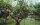Fifteen lumberjacks would take 12 days to destroy the broken trees. How many days would this work require if 5 workers added to it after 4 days?
2. Two citiesThe distance between cities A and B is 132 km. At 9.00 am, the cyclist started the bike at an average speed of 24 km/h, and at 10.00 h started from the B cyclist at an average speed of 30 km/h. How long and far from A will they both meet?
3. The cruise ship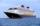The cruise ship has a speed of 12 km / h at a calm surface. When we sail 45 km along the river and 45 km back, it took us exactly 8 hours. Which (constant) speed of flow of the river?
4. Electric energyThere are 875 identical light bulbs in the sports hall lighting for 2 hours. How long does the same amount of electricity consume 100 such bulbs?
5. Two trainsThrough the bridge, long l = 240m, the train passes through the constant speed at time t1 = 21s. A train running along the traffic lights at the edge of the bridge passes the same speed at t2 = 9s. a) What speed v did the train go? b) How long did it tak
6. Three pumps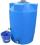The water tank is emptied with the first pump in 6 hours, with the second pump in 2 hours. In order to be emptied in 1 hour, all three pumps had to be started at once. How long will take tank be emptied by only the third pump?
7. Painters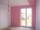Six of the painters paint 90 m of fence in five hours. For how long would the 4 painters paint a 45 meter fence? How many meters fence painted painters 5 for two hours?
8. TrianglesIvo wants to draw all the triangles whose two sides of which have a length of 4 cm and 9 cm and the length of the third side is expressed in whole centimeters. How many triangles does he have?
9. Fruit juice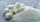Fruit juice contains 37.5% sugar. How many percents of sugar will be in the flavored mineral that we prepare from 100 grams of fruit juice and 1.4 liters of mineral? (1 liter = 1 kg)
10. Inter city busThe inter city bus leaves Suva at 10.00am and reaches Nadi at 1.00pm covering a distance of 219km. How long did it take to reach the bus Nadi?
11. The tourist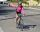The tourist came started from the hostel at an average speed of 5km/h. Half an hour later, the bicyclist started along the same route at a speed of 20km/h. How many minutes will a cyclist catch up and how many kilometers will he go?
12. Inlet and outletThe pool has a capacity of 50 hl. The inlet pipe flows in 1 minute 1.25 hl, and the waste pipe outlet is draining the full pool in 50 minutes. How long will take the empty the full pool when both the inlet and outlet are opened at the same time?
13. Two workersThe first worker would himself completed the work in 8 hours, the other in 6 hours. After two hours of joint work left the first worker to a doctor and other completed work himself. How many hours worked the other worker himself?
14. WorkThe first worker would need less than 4 hours to complete the task than the other worker. In fact, both workers worked for two hours together, then the first worker did the remaining work himself. In what proportion should the remuneration of the workers b
15. The tank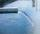The tank had 9 inflows to be filled in 21 days. After 9 days, 3 trips out. How many days did the remaining 6 tributaries fill the tank?
16. Tourist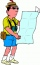A tourist walked an average speed of 3.5 km/h route in 6 hours. Calculate how many hours he would have passed at an average speed of 5.5 km/h.
17. Four paversFour pavers would pave the square in 18 days. How many pavers do you need to add to done work in 12 days?
18. MO C–I–1 2018An unknown number is divisible by just four numbers from the set {6, 15, 20, 21, 70}. Determine which ones.
19. Resistance of the resistorThe resistor terminals have a voltage of 20 V and a current of 5 mA is passed through. What is the resistance of the resistor?
20. Flowerbed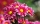Ondra digs the bed in 20 minutes. Cuba in 30 minutes. For how long do they dig together?

Do you have an interesting mathematical word problem that you can't solve it? Enter it, and we can try to solve it.

To this e-mail address, we will reply solution; solved examples are also published here. Please enter the e-mail correctly and check whether you don't have a full mailbox.

Please do not submit problems from current active competitions such as Mathematical Olympiad, correspondence seminars etc...